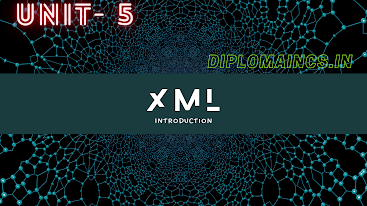## 10/20/21

### Java 2020 question

JAVA

Sub Code: - 1618505

2020 (ODD)

Time : 3Hrs

Semester  V(New)/CSE

JAVA

Full Marks : 70

Pass marks : 28

Group A

Choose the most suitable answer from the following options: -   (1*20=20)

(i) Which of the following is not OOPS concept in JAVA?

(a) Inheritance

(b) Encapsulation

(c)  Polymorphism

(d)  Compilation

(ii) Which of these keywords is used to make a class?

(a) Class

(b) Struct

(c) Int

(d) None of the mentioned

(iii) What is the return type of a method that does not return any value?

(a) Int

(b) Float

(c) Void

(d) Double

(iv) Method having same name as its class name is:

(a) Struct

(b) Enum

(c) Constructor

(d) Argument

(v) Which method can be defined only once in a program?

(a) Main method

(b) Finalize method

(c) Static method

(d) Private method

(vi) Size of an integer can be:

(a) -231 to 231 - 1

(b) 0 to -2 16

(c) -28 to 28 - 1

(d) -215 to 215 -1

(vii) Which of the following assignment operator doesn’t exist in JAVA?

(a) >>

(b) %=

(c) >>>

(d)<<

(viii) Which loop is always executed once:

(a) For

(b) For each

(c) Do … While

(d) While

(ix) Which of the following is not valid flow control statement?

(a) Break ;

(b) Continue outer;

(c) Return;

(d) Exit ()

(x) A top level class may have which one of the following access modifiers?

(a) Package

(b) Private

(c) Protected

(d) Public

(xi) A subclass is also called as:

(a) Inner class

(b) Nested class

(c) Derived class

(d) Hidden class

(xii) Which of the following is not a wrapper class?

(a) Vector

(b) Character

(c) Boolean

(d) Integer

(xiii) A default constructor has:

(a) No arguments

(b) Has no return type

(c) Has one argument but no return type

(d) Has two arguments

(xiv) Math class belongs to the package:

(a) java.,math

(b) java.lang

(c) java.util

(d) None of these

(xv) A package is a collection of:

(a)  Classes

(b) Interfaces

(c)  Editing tools

(d) Class and interfaces

(xvi) Primary purpose of inheritance is:

(a)  Code reuse

(c)  Overriding

(d) None of the above

(xvii) Which method/ methods cannot be overridden?

(a)  Static

(b) Final

(c)  Only (a)

(d) Both (a) and (b)

(xviii) Which of the following does not deal with exceptions?

(a) Throws

(b) Throw

(c)  Finalize

(d) Finally

(xix) What is the default priority of a thread in java?

(a)  10

(b) 5

(c)  3

(d) 1

(xx) The class at the top the AWT hierarchy is

(a) Component

(b) Window

(c) Container

(d) Frame

Group:-"B"

Answer all Five Questions: -                         (5*4=20)

2.

a.          What is the difference between Java and Java script?\

b.          What is JVM?

OR

a.          What are primitive data types in Java?

b.          Java is both compiled and interpreted. Explain in brief.

3.

a.          What is a class in Java? Give one example.

b.          What do you mean by scope and lifetime of a variable?

OR

a.          What is meant by objects in Java?

b.          Explain the casting of variables with an example.

4.

a.          What is the difference between for loop and while loop?:

b.          What is a ternary operator?

OR

b.          What do you mean by constructors?

5.

b.          What do you mean by constructors?

OR

a.          What is meant by polymorphism in Java?

b.          What is package in Java?

6.

a.     Explain in brief the try catch blocks.

b.     What is meant by garbage collection in Java?

OR

a.          What is meant by Exception handling in Java?

b.          What is the use of final block?

Group:- "C"

Answer all Five Questions: -                         (5*6=30)

7.  What is the meaning of inheritance in Java? Describe various types of inheritance in Java.

OR

Explain in brief multithreaded programming. Also describe Alive(), joint(), suspend() and resume().

8. Define Applets and its life cycle.

OR

Explain any two streams in Java. Write a short program to accept two numbers from the user and display their sum.

9. What is difference between an interface and abstract class?

OR

What do you mean by a package? How is it different from an interface and how an interface and how can we import them?

10. Write short notes on:

a.    Wrapper class

b.    File input stream

c.     Buffered input stream

OR

What is enumeration interface? Explain in brief. What is vector in Java? Explain in brief.

11. Write a program to find the Fibonacci series. State differences between call by value and call by reference.

OR

Write a program to swap two numbers using call by reference. Explain call by reference with an example.